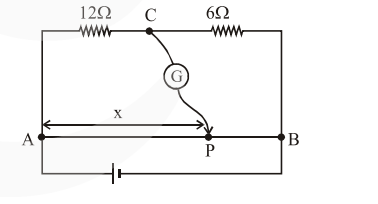# Consider a 72 cm long wire AB as shown in the figure.`
Question:

Consider a $72 \mathrm{~cm}$ long wire $\mathrm{AB}$ as shown in the figure. The galvanometer jockey is placed at $P$ on $A B$ at a distance $x \mathrm{~cm}$ from $A$. The galvanometer shows zero deflection.The value of $x$, to the nearest integer, is

Solution:

In Balanced conditions

$\frac{12}{6}=\frac{x}{72-x}$

$x=48 \mathrm{~cm}$